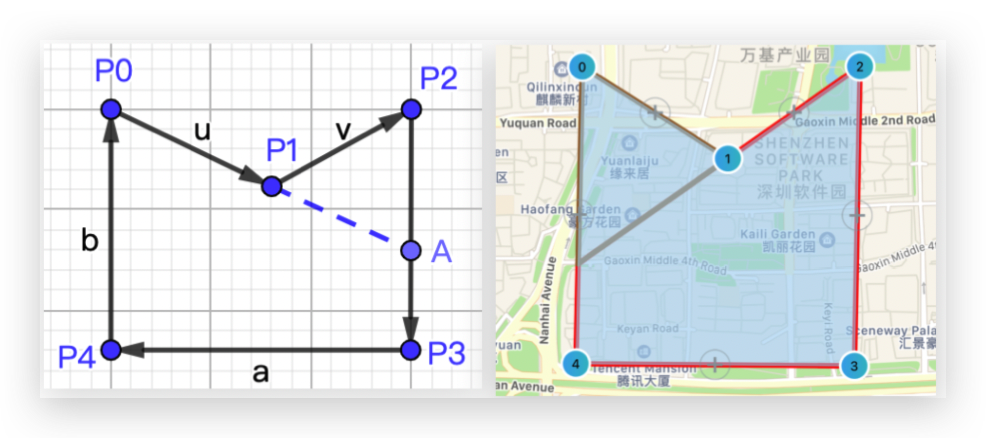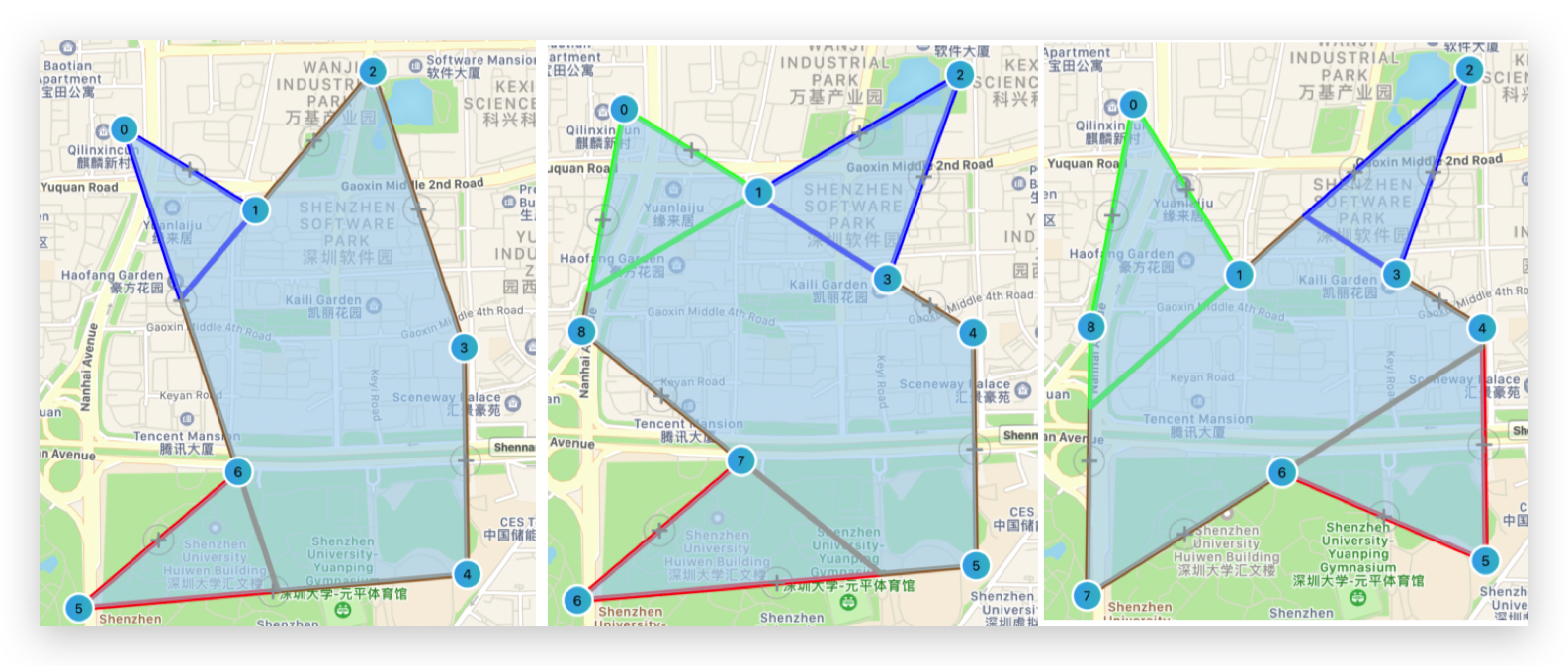# 如何切割凹多边形

## 向量方法

### 1. 找出异号的边向量叉积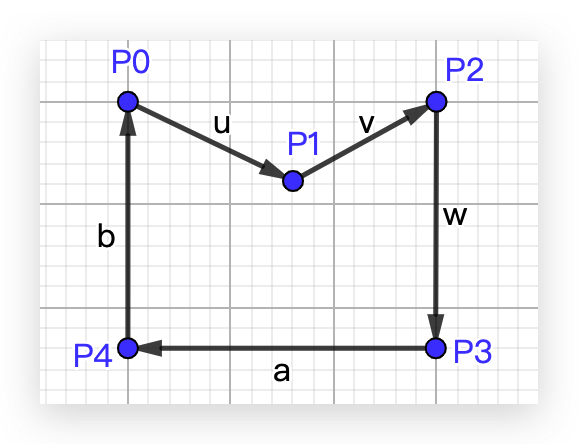$$\vec{u} \times \vec{v} > 0 \ \vec{v} \times \vec{w} < 0 \ \vec{w} \times \vec{a} < 0 \ \vec{a} \times \vec{b} < 0 \$$

### 2. 延长异号边进行切割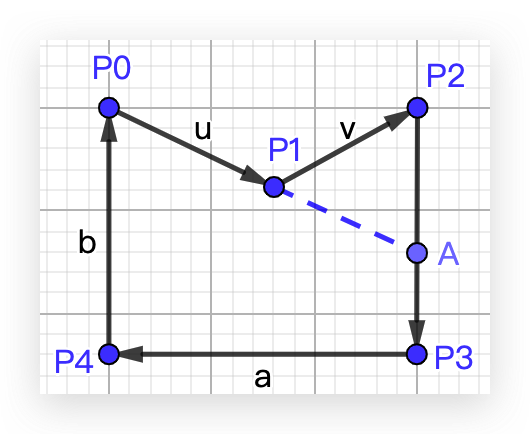1. 如何找到交点位置，即交点所在的边和交点的坐标。
2. 得到交点坐标后，如何分割原数组，且得出点顺序是正确的两个多边形数组。

### 3. 找到交点位置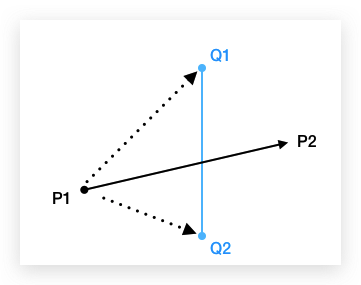### 4. 分割原数组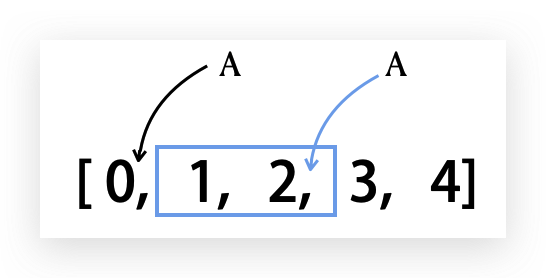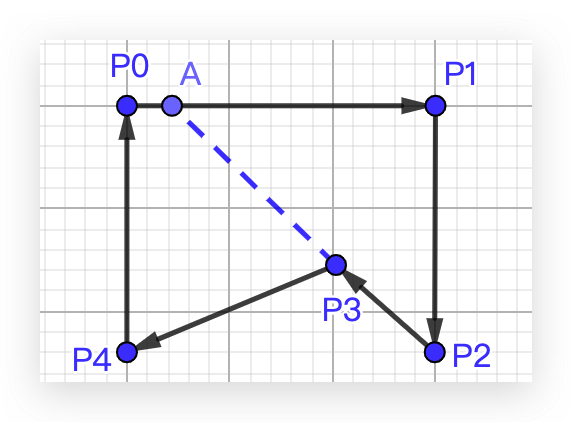### 5. 注意坐标系